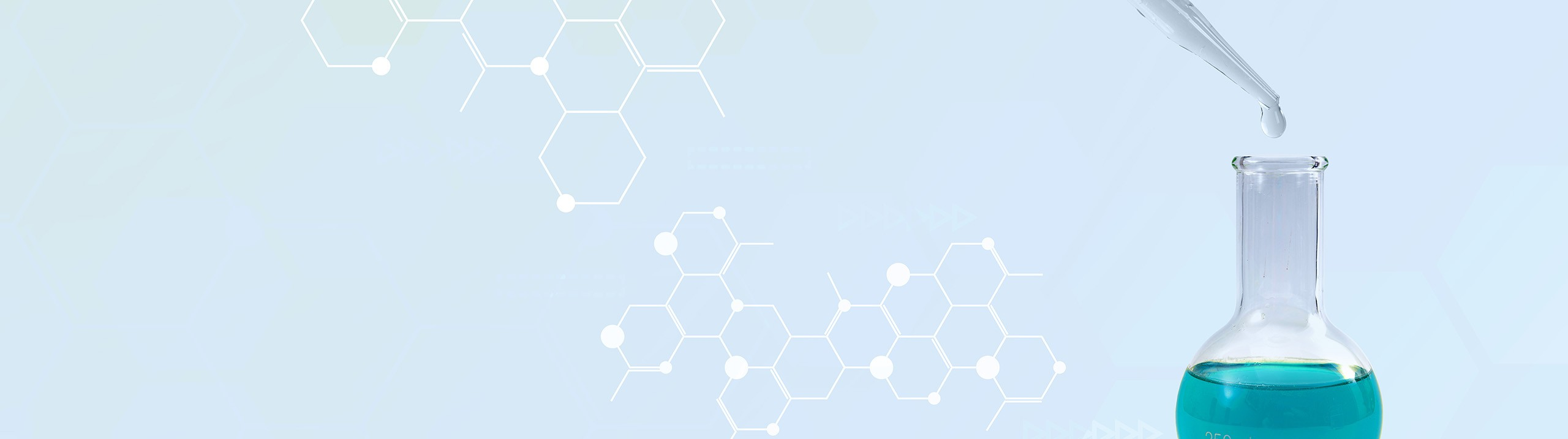Start typing, then use the up and down arrows to select an option from the list.# Analytical Chemistry

Learn the toughest concepts covered in your Analytical Chemistry class with step-by-step video tutorials and practice problems.

Chemical Equilibrium

The pH scale is useful in converting very small concentration values into more manageable numbers.

## pH and pOH

1

#### concept

pH and pOH5m
Play a video:
2

#### example

pH and pOH2m
Play a video:

## pH and pOH Calculations

3

#### example

pH and pOH Calculations 13m
Play a video:
here it states of the following options, a solution with which ph would have the greatest concentration of hydro ni um ions On the previous page we said that if we increased our ph concentration that would lead to a drop in the amount of H plus ions available here. We want the opposite, we want the greatest amount of H plus ions available. So we would look for the lowest ph so the lowest ph would translate into the highest amount of H plus ions and the lowest amount of hydroxide ions. So for this question it's simply option four. Now remember we're dealing with log function here. So if you're comparing a ph of four to a ph of five, just realize here that increasing ph by one unit means a tenfold increase for O H minus concentration. And it would mean a tenfold decrease. Mhm. For H plus concentration in the opposite way, If you went from PH equals four, two P. H equals let's say two. So every one unit um that we move up and down the ph scale, that's a tenfold increase. Or decrease for H plus or oh minus concentration here we're going to ph of two. So we're becoming more acidic. So that means we're increasing the amount of H plus concentration. But here we're moving it moving down by two units, every ph unit we go down we increase H plus tenfold since we're moving here down by two units that be 10 times 10. So that would be 100 fold increase for H plus concentration and 100 fold decrease for O H minus concentration. So this question itself was simple here. I added a couple more things to it. Just remember when you're going on the ph scale, moving up and down the ph scale by units of one that that translates to a 10 fold increase or decrease to either your H. Plus concentration or your O. H minus concentration. Use the two examples I gave here as basically a road map to see what happens to their concentration as we go up and down the ph scale. Now that we've attempted this one, try to do example to hear HBR is a strong acid. So we have to understand that in order to find its true mass at the end. So attempted on your own. But if you get stuck, come back and see how I approach that same exact question.
4

#### example

pH and pOH Calculations 14m
Play a video: